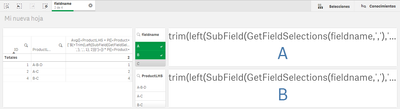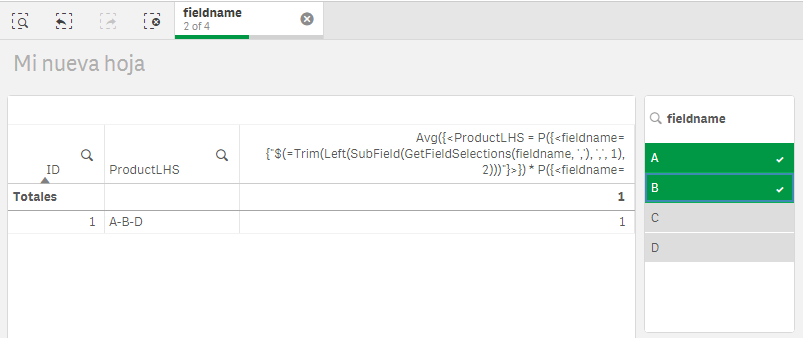# App Development

Announcements
April 22, 2PM EST: Learn about GeoOperations in Qlik Sense SaaS READ MORE
cancel
Showing results for
Did you mean:Partner

## How to use many selections of a field to filter another field.

Hi,

I've done a model with Association Rules with my Field Products {A, B, C, D, E}.

So, for exemple i've obtained the rule  {A,C}-->{E} that means that if you have bought A and C, normally you will also buy the E.

In Qlik the table is like:The relationship between them is: if a click on Product 'A' the result in table must be rows with 'A-B-D' and 'A-C' because they contain 'A'. That works good. The problem is that my goal would by to click in more than one product and obtain only the rules with this products in the same row. For example, if I click on 'A' and 'B' of Products, the only row that should be shown is first one: A-B-D. But qlik by default selects the rowns tha contain 'A' or 'B', like 'A-B-D', 'A-C' and 'B-C'.

I've got the objetive manually with:

Avg({<ProductLHS = P({<Product={'A'}>})*P({<Product={'B'}>})>} ID)

But I need that this filter with 'A' or 'B' depend on selections of Product. With

trim(left(SubField(GetFieldSelections(fieldname,','),',',1),2))

trim(left(SubField(GetFieldSelections(fieldname,','),',',2),2))

I obtain the selections, but when I introduce it on the Set Analysis of measure it doesnt recognize the selections.

Does anybody know how to fix it? Thanks!!

Labels (3)

• ### qliksense filter

1 Solution

Accepted SolutionsMVP

I would simplify the expression to this

``````Avg({<ProductLHS = P({<fieldname={"\$(=If(Len(Trim(SubField(GetFieldSelections(fieldname,','),',',1))) = 0, '*', Trim(SubField(GetFieldSelections(fieldname,','),',',1))))"}>}) *
P({<fieldname={"\$(=If(Len(Trim(SubField(GetFieldSelections(fieldname,','),',',2))) = 0, '*', Trim(SubField(GetFieldSelections(fieldname,','),',',2))))"}>}) *
P({<fieldname={"\$(=If(Len(Trim(SubField(GetFieldSelections(fieldname,','),',',3))) = 0, '*', Trim(SubField(GetFieldSelections(fieldname,','),',',3))))"}>}) *
P({<fieldname={"\$(=If(Len(Trim(SubField(GetFieldSelections(fieldname,','),',',4))) = 0, '*', Trim(SubField(GetFieldSelections(fieldname,','),',',4))))"}>})
>} ID)``````

Basically checking for each selection if there is a selection... if there is no selection replace the Product with * which means include everything....

Note: * will exclude nulls in fieldname... so make sure there are no nulls....

8 RepliesMVP

Are you saying that this doesn't work for you?

``Avg({<ProductLHS = P({<Product={"\$(=Trim(Left(SubField(GetFieldSelections(fieldname, ','), ',', 1), 2)))"}>}) * P({<Product={"\$(=Trim(Left(SubField(GetFieldSelections(fieldname, ','), ',', 2), 2)))"}>})>} ID)``Partner
Author

Yes,and if I put  Avg({<ProductLHS = P({<fieldname={'A'}>})*P({<fieldname={'B'}>})>} ID) it works:MVP

What is the different between fieldname and Product? Are you able to share a sample qvf file to check this out?Partner
Author

Oh Sorry,

fieldname and Product are the same.

I put here my example app.

Thanks.MVP

This seems to work

``Avg({<ProductLHS = P({<fieldname={"\$(=Trim(Left(SubField(GetFieldSelections(fieldname, ','), ',', 1), 2)))"}>}) * P({<fieldname={"\$(=Trim(Left(SubField(GetFieldSelections(fieldname, ','), ',', 2), 2)))"}>})>} ID)``

Only change that I made was to change the field name Product to fieldname here...Partner
Author

Yes! It works!

So finally, if I want that show only cases based on the four posible selections in this case, how do I have to do it?

For example,

• No selections: show me all possible values
• One filter: show me case with this value
• Two filters: show me cases with both values
• Etc

I mean, extend this to all possible cases. I'm testing with:

if(GetSelectedCount(fieldname)=1,
Avg({<ProductLHS = P({<fieldname={"\$(=Trim(Left(SubField(GetFieldSelections(fieldname, ','), ',', 1), 2)))"}>})>} ID),
if(GetSelectedCount(fieldname)=2,
Avg({<ProductLHS = P({<fieldname={"\$(=Trim(Left(SubField(GetFieldSelections(fieldname, ','), ',', 1), 2)))"}>}) * P({<fieldname={"\$(=Trim(Left(SubField(GetFieldSelections(fieldname, ','), ',', 2), 2)))"}>})>} ID),
if(GetSelectedCount(fieldname)=3,
Avg({<ProductLHS = P({<fieldname={"\$(=Trim(Left(SubField(GetFieldSelections(fieldname, ','), ',', 1), 2)))"}>}) * P({<fieldname={"\$(=Trim(Left(SubField(GetFieldSelections(fieldname, ','), ',', 2), 2)))"}>})* P({<fieldname={"\$(=Trim(Left(SubField(GetFieldSelections(fieldname, ','), ',', 3), 2)))"}>})>} ID),
if(GetSelectedCount(fieldname)=4,
Avg({<ProductLHS = P({<fieldname={"\$(=Trim(Left(SubField(GetFieldSelections(fieldname, ','), ',', 1), 2)))"}>}) * P({<fieldname={"\$(=Trim(Left(SubField(GetFieldSelections(fieldname, ','), ',', 2), 2)))"}>})* P({<fieldname={"\$(=Trim(Left(SubField(GetFieldSelections(fieldname, ','), ',', 3), 2)))"}>})* P({<fieldname={"\$(=Trim(Left(SubField(GetFieldSelections(fieldname, ','), ',', 4), 2)))"}>})>} ID)
))))

but it doesn't work....MVP

I would simplify the expression to this

``````Avg({<ProductLHS = P({<fieldname={"\$(=If(Len(Trim(SubField(GetFieldSelections(fieldname,','),',',1))) = 0, '*', Trim(SubField(GetFieldSelections(fieldname,','),',',1))))"}>}) *
P({<fieldname={"\$(=If(Len(Trim(SubField(GetFieldSelections(fieldname,','),',',2))) = 0, '*', Trim(SubField(GetFieldSelections(fieldname,','),',',2))))"}>}) *
P({<fieldname={"\$(=If(Len(Trim(SubField(GetFieldSelections(fieldname,','),',',3))) = 0, '*', Trim(SubField(GetFieldSelections(fieldname,','),',',3))))"}>}) *
P({<fieldname={"\$(=If(Len(Trim(SubField(GetFieldSelections(fieldname,','),',',4))) = 0, '*', Trim(SubField(GetFieldSelections(fieldname,','),',',4))))"}>})
>} ID)``````

Basically checking for each selection if there is a selection... if there is no selection replace the Product with * which means include everything....

Note: * will exclude nulls in fieldname... so make sure there are no nulls....Partner
Author

Yes, this is the finally solution.

Thank's a lot Sunny 🙂  You're the real MVP!!!!!!